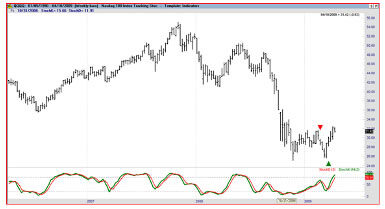## What are Stochastics?

This is a momentum indicator that shows the location of the current close relative to the high/low range over a set number of periods.

Momentum indicators try to identify turning points by measuring how fast prices are rising or falling.

## How Do Stochastics Work?

The calculation of the stochastic can be confusing.

First a value known as %K is calculated with this formula:

%K = 100*((C-L14) / (H14-L14))

C is the most recent closing price; L14 is the lowest price in the last fourteen periods; and H14 is the highest price in the last fourteen periods. We used 14 as an example, but any number can be used here.

A 3-period moving average of %K is then calculated and called %D (no one really knows where the names came from, but they are widely used in almost all software packages). A buy signal is given when %K crosses above %D and sell signals occur when %K falls below %D.

An example is shown in the image below.Image: The stochastics indicator offers clear trading signals. Only one trade is highlighted in this chart, but there are more than a dozen signals shown.

For stochastics, readings below 30 are considered oversold and you would only take buy signals if the indicator is below that level. A value of 70 is considered overbought and sell signals occurring below that level would be ignored. This leads to longer trades and should result in fewer losses.

## Why Do Stochastics Matter?

One problem with using indicators to generate trading signals is that there are a large number of losing trades. One way to improve the odds of a winning trade is to ignore signals unless the market is at an oversold or overbought extreme.